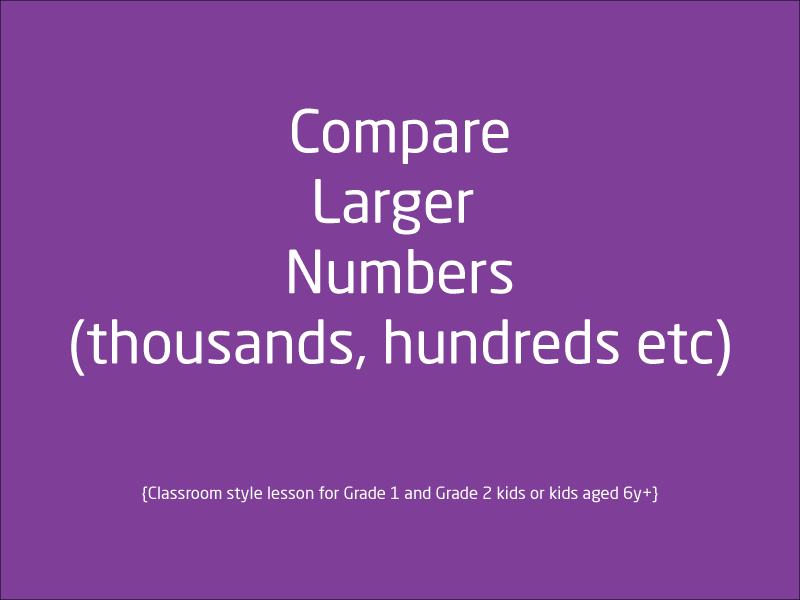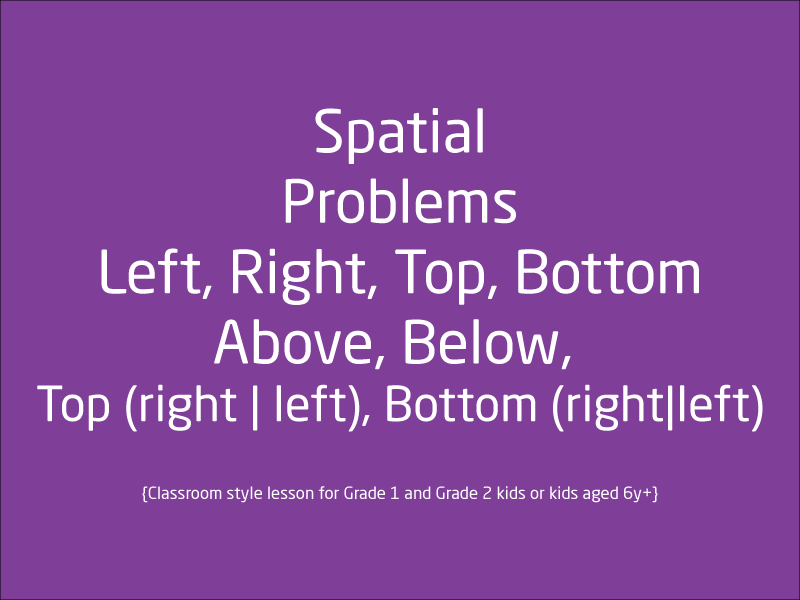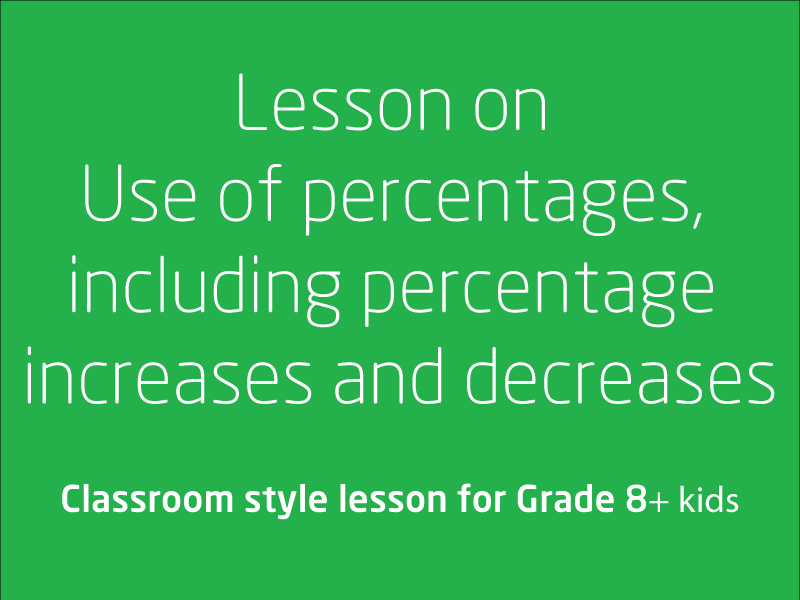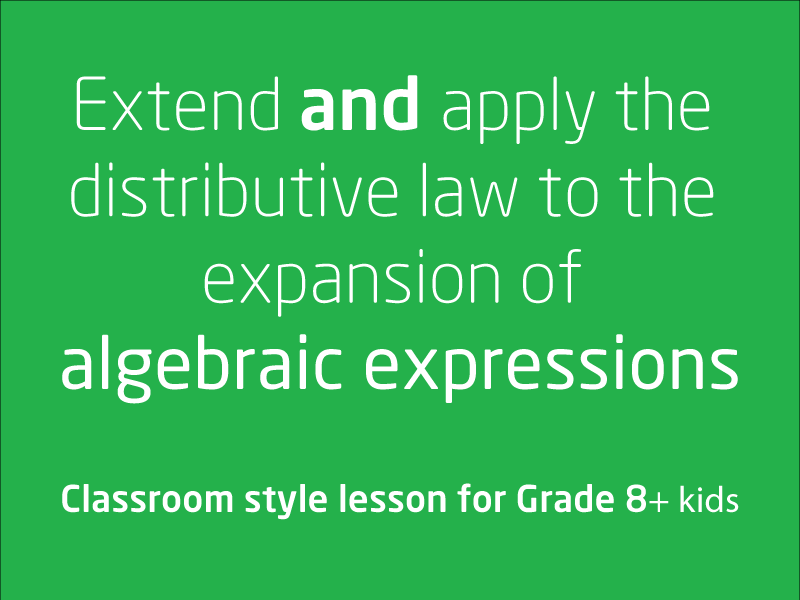# Free video lessons for Foundation to Grade 8 students### Comparing numbers: Thousand place value

This lesson focus on comparing larger number and have got one example of comparing decimal point numbers as well. This classroom-style lesson was recorded live by the tutor. This l...

1 videos
Basic difficulty
0 0 0### The Spatial Problems

This Lesson builds up from where the previous lesson on spatial problem left. This Lesson covers positions such asTopLeftRightBottomAboveBelowTop LeftTop RightBottom LeftBottom Rig...

1 videos
Basic difficulty
0 0 0### Lesson on rates and ratios, with and without digital technologies

This video lesson is a classroom style lesson where you rates and ratios are discussed in length. You should aim to achieve understanding that rate and ratio problems can be s...

2 videos
Intermediate difficulty
0 0 0### Understanding and Evaluating Exponents

In this video lesson we will Investigate index notation and represent whole numbers as products of powers of prime numbers.We aim that kids will achieve the following in this ...

2 videos
Intermediate difficulty
0 0 0### Investigate terminating and recurring decimals

Students would be able to recognise terminating, recurring and non-terminating decimals and choosing their appropriate representations after taking this lesson

1 videos
Intermediate difficulty
0 0 0### Investigate the concept of irrational numbers, including π (Pi)

In this video lesson kids will firm their understanding on real number system includes irrational numbers. Tutor will also discuss special numbers such as Pi (π)

1 videos
Intermediate difficulty
0 0 0### Lesson on Use of percentages, including percentage increases and decreases

This is a two part series, at the end of these lesson we would like kids to have basic understanding of percentages, download the related worksheets, attempt few quizzes to get a f...

2 videos
Intermediate difficulty
0 0 0### Understanding profit and loss

This video lesson touch base on explaining profit and loss, several examples are provided. We recommend to watch this lesson as many times, because we think this concept is ve...

2 videos
Intermediate difficulty
0 0 0### Extend and apply the distributive law to the expansion of algebraic expressions

This video lesson concentrates around introducing you to extending and applying the distributive law to the expansion of algebraic expressions. This ia a multi part video less...

2 videos
Intermediate difficulty